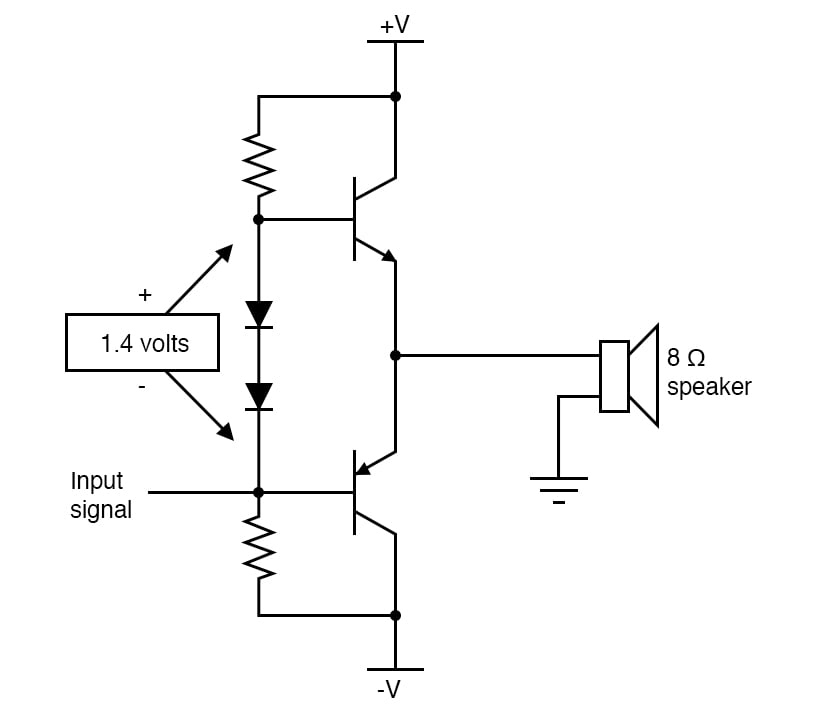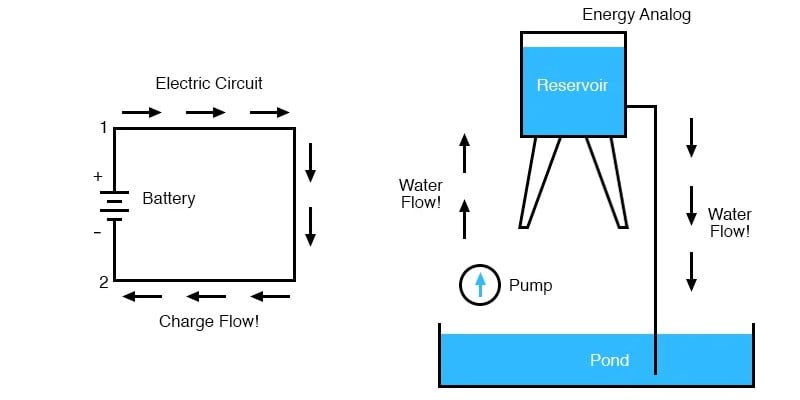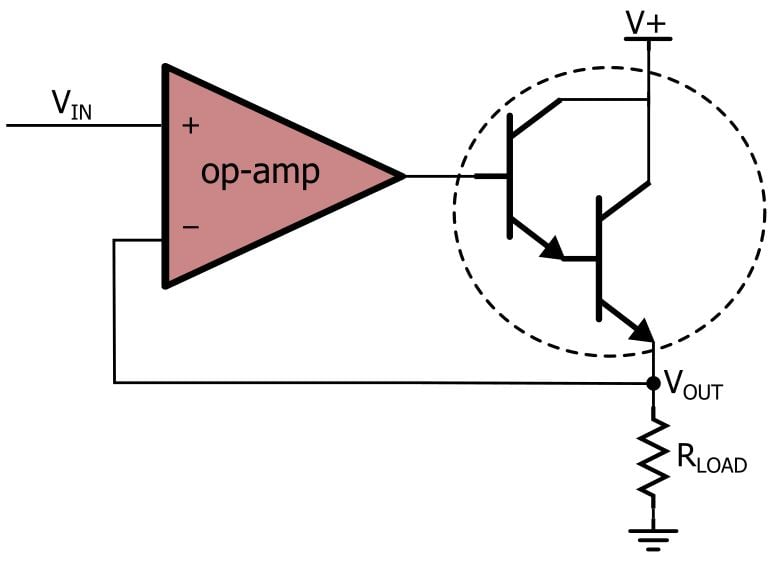Technical Article

# Can Voltage Be Negative? Understanding Negative Voltage

April 02, 2023 by Robert Keim

## In addition to explaining the nature of a negative voltage, this article briefly discusses how negative voltages are generated and why they are useful in circuit design.

The concept of negative voltage is sometimes less intuitive than the concept of positive voltage. Perhaps this is because many low-voltage electronic systems do not use negative voltage supplies or because a “negative” voltage implies that a source has a “less than zero” ability to drive current through a circuit. Though many useful and even high-performance devices can be designed and implemented without negative voltages, understanding negative voltage is key to understanding voltage in general, and most people who work with electronics will eventually encounter a circuit that requires a negative-voltage power supply (Figure 1).### A Quick Review of Voltage

The word “voltage” is so common now, in both technical and everyday contexts, that it’s a good idea to occasionally remind ourselves what voltage really is from a scientific perspective.

Electric charge can do work in the world, and work is analyzed and quantified using the scientific concept of energy. If charged particles are moving through wires and, for example, causing a motor to rotate, they have energy and are actively doing work. Voltage is electrical energy, but it is not an active type of energy. Voltage tells us the capacity of charged particles to do work, given the proper circumstances—in other words, voltage is a form of potential energy. More specifically, it is the amount of electric potential energy (measured in joules) per coulomb of electric charge.

However, this definition is still incomplete since a voltage cannot exist in isolation. When an electric charge starts doing work, it moves from one location to another, and similarly, we must measure the voltage as the potential energy (per unit charge) of one location relative to the potential energy (per unit charge) of some other location. Thus, voltage is always a differential measurement. When we say that at some point, a circuit “is at five volts,” what we really mean is five volts relative to the circuit’s assumed zero-volt reference point.

To help understand voltage, Figure 2 shows an example diagram of an electric circuit and charge flow, as well as a useful water analogy.### What is Negative Voltage?

A negative voltage is not fundamentally different from a positive voltage; both represent potential energy relative to a reference potential. If a circuit node is at a positive voltage relative to the reference node and when we connect these two nodes with a conductor, conventional current will flow from the positive node to the reference node. If a circuit node at negative voltage is connected to the reference node, conventional current will flow from the reference node to the negative node. Keep in mind that, in low-voltage electronic design, the reference node is typically called “ground,” but something like “circuit common” would be more accurate.

To better wrap your head around this concept, I think that an altitude analogy would be helpful here. Let's say that Mount Everest is 29,032 feet tall, but when reported in isolation, this number is actually meaningless. What we really mean is that the peak of Mount Everest is 29,032 feet above sea level. Sea level is defined as having an altitude of zero—i.e., it’s the reference altitude. The lowest land altitude on earth, corresponding to the surface of the Dead Sea, is about 1,400 feet below sea level, which we could describe as an altitude of negative 1,400 feet.

The difference between a positive and negative altitude is their position relative to the reference point for zero altitudes, just as the difference between a positive and negative voltage is their electrical “position” relative to the zero-volt reference node. If we used the bottom of the Mariana Trench as a reference point, both Everest and the Dead Sea would have positive altitudes. If we used the upper edge of the stratosphere as a reference point, both altitudes would be negative. Likewise, we could “change” a positive voltage to a negative voltage, or vice versa, by generating a new voltage and using it as the zero-volt reference point.

The altitude comparison is particularly apt because the effect of gravity is analogous to the effect of electric potential energy. A ball on Mount Everest will roll toward sea level, and a ball at sea level will roll toward the shore of the Dead Sea. Similarly, a positive voltage causes conventional current to flow from the positive node to the reference node, and a negative voltage causes current to flow from the reference node to the negative node.

### Generating Negative Voltages

Rudimentary circuits often begin with an unregulated power supply, for example, from a battery or wall transformer, that is reduced to 5 V or 3.3 V using a linear regulator. We cannot use this method to generate a negative voltage—not because negative voltages are fundamentally different from positive voltages, but because linear regulators function by dissipating energy. To convert positive voltage to negative voltage, we can use regulator circuitry that also stores energy.

Capacitors and inductors are basic electronic components that can store energy, and both can be used to generate negative voltages. Capacitor-based negative voltage generators belong to the “charge pump” category of power-supply circuits, and inductor-based negative voltage generators belong to the “switch mode” category. Inductor-based solutions, which are also called DC/DC converters and switching power supplies, are much more common.### A Negative Voltage Application and Design Resources

Though many electronic devices accomplish their required functionality without a negative supply voltage, some applications significantly benefit from the presence of a “bipolar” power supply, i.e., a power supply with both positive and negative voltage rails. For example, negative voltages allow sinusoidal signals to extend above and below zero, just as a theoretical sinusoid does, and high-power amplifiers with a bipolar supply don’t need a (potentially expensive) DC-blocking capacitor.• O
olaney April 07, 2023

Not sure if I need this e-newsletter subscription if it keeps serving up the equivalent of introductory high school electronics.

Like.
• M
MisterBill2 April 07, 2023

Really, while I do not intend to be hostile,  this discussion is suitable for a seventh grade science class back about 1962.
The answer is far simpler: It all depends on which way you look at it. Standing at the positive terminal of a battery, the other terminal is indeed negative.
Like all vectors, voltage has both magnitude and direction.

Like.